# Resonant Layer Equations

In a conventional tokamak plasma, the Lundquist number,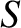, which is the nominal ratio of the plasma inertia term to the resistive diffusion term in the plasma Ohm's law , is very much greater than unity. In fact, according to Table 1.5,typically exceeds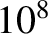in a tokamak fusion reactor. However, a resonant layer is characterized by a balance between plasma inertia and resistive diffusion . Such a balance is only possible if the layer is very narrow in the radial direction (because a narrow layer enhances radial derivatives, and, thereby, enhances resistive diffusion). Let us define the stretched radial variable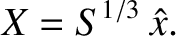(5.56)

Assuming that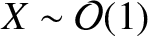in the layer (i.e., assuming that the layer thickness is roughly of order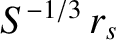), and making use of the fact that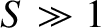, we deduce that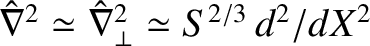. Hence, the linear equations (5.39)–(5.42) reduce to the following set of resonant layer equations [7,15]: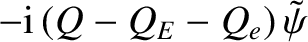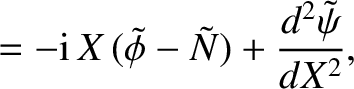(5.57)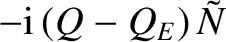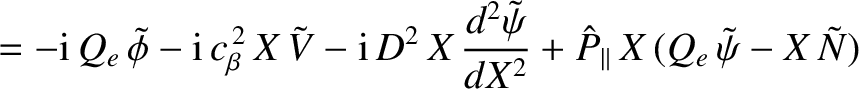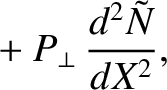(5.58)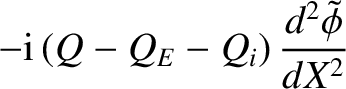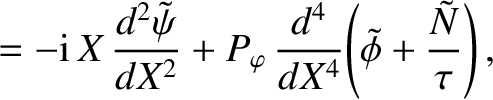(5.59)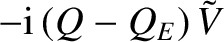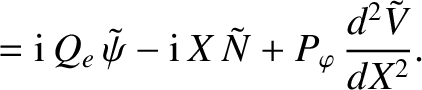(5.60)

Here,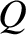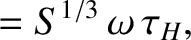(5.61)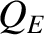(5.62)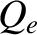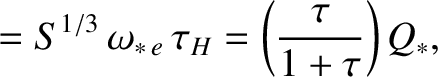(5.63)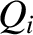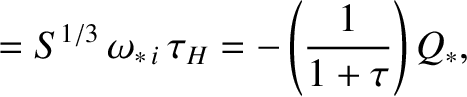(5.64)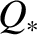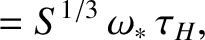(5.65)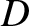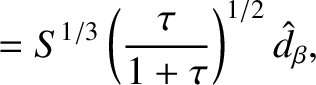(5.66)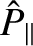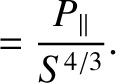(5.67)

Table: 5.1 Dimensionless parameters appearing in resonant layer equations for a low-field and a high-field tokamak reactor. See Equations (4.5), (5.65), (5.66), (5.53), (5.54), and (4.65).
 Low-Field High-Field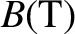5.0 12.0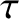1.0 1.0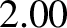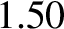3.02 2.26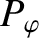874 874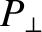287 2870.635 0.635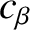0.128 0.128

Table 5.1 gives estimates for the values of the dimensionless parameters that characterize the resonant layer equations, (5.57)–(5.60), in a low-field and a high-field tokamak fusion reactor. (See Chapter 1.) These estimates are made using the following assumptions: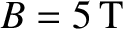(low-field) or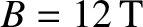(high-field),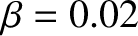,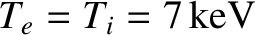,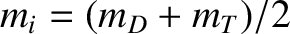(where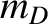and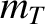are the deuteron and triton masses, respectively),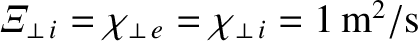,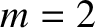,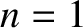,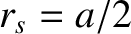(where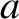is the minor radius of the plasma),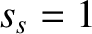,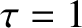,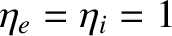, and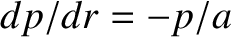. The parallel energy diffusivities,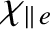and, are estimated from Equations (2.319) and (2.320), respectively, using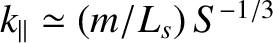, which is the typical parallel wavenumber of the tearing perturbation at the edge of a resistive layer whose characteristic thickness is. Note that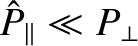, which allows us to neglect the parallel transport terms (i.e., the terms involving) in Equation (5.58). The neglect of these terms is justified because the linear layer width is much less than the critical width,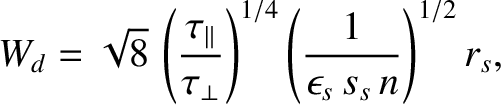(5.68)

below which parallel transport is unable to constrain the perturbed electron temperature to be constant along magnetic field-lines .